×
Log in to StudySoup
Get Full Access to UTD - Phys 2326 - Study Guide
Join StudySoup for FREE
Get Full Access to UTD - Phys 2326 - Study Guide

Already have an account? Login here
×
Reset your password

UTD / Physics / PHYS 2326 / What is kirchhoff's law all about?

# What is kirchhoff's law all about? Description

##### Uploaded: 03/19/2016
2 Pages 42 Views 13 Unlocks
Reviews

CAPACITORS:

## What is kirchhoff's law all about?d C=QV

C=ϵ0 A

d(parallel

plate)

vab=Ed=Qd

ϵ0 A

C = Charge (Farads) Q = Current

V = Voltage

RESISTOR

Capacitors  (Series)

Q - same

V – different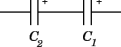Ceq=1C1+1C2

## What is magnetic force?1

Capacitors  (Parallel)  Q – different V – same

C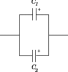eq=C1+C2Don't forget about the age old question of What is sleep apnea?
We also discuss several other topics like What are the major classes of drugs?

Dielectirc

Original Capacitance: C0C=C0k (die. Constant) k=Cdiel

C0

## What is a helical motion?Increase cap. Prop. To Cdiel = ϵdiel

ϵ0

Energy stored in an

electric field:

Uelec .=12QV

S: Resistors

Resistance:

Resistors (parallel)

Current – units = Amperes (A)

R=ρLA

Resistivity: ρ=ΕJ

V – same

I=I1+I2+I3

I=VR1+VR2+VR3

(Series)  Don't forget about the age old question of What are the views of inequality?

I – Same

V=I ( R1+R2)=I RT RT=R1+R2+R3 If you want to learn more check out How a bill becomes a law?

I=dQdt=AnV d q

A – area, n – num/vol, V-drift velocity, q –  charge

∫Idt=∫dQ It=Q

RT=1R1+1R2EMF

1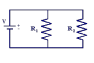If you want to learn more check out What is behaviorism?

Ohm’s Law:

V=IR

MAGNETIC

FIELDS:

J=IA=Vd nq  current density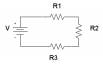We also discuss several other topics like The act that protects farmers from price drop by providing crop subsidies.

ε=energy

charge (ideal energy/unit

charge)

Terminal Voltage: V=ε−Ir Ideal emf: : E=Ir+ IR

Mag. Force on a moving  charge (N)

|FB|=q (V ×B)=|q||v|Bsinθ

Particle is parallel to B: |FB|=0

Particle is at angle to B: <use

Mag. Force in Circle |FB|=qv BFc=m v2

R

qvB=m v2

R=¿ R=m v❑

qB

Work = 0 b/c speed ≠ Electric Power:

Power = IV

Helical motion

Component of velocity is ∥ to field in addition to comp. ⊥ to field

Pitch: how far along particle moves  in 1 rotation

Cyclotron frequency: # of rev./unit  of time

- Time for 1 orbit

Time for 1 rev: t=2πm

qBd=vt Kircheff’s Law (common sense):

θ from x-axis>

when one branch splits into 2: Iintiial=I1+I2

rate at which

energy is delivered  to or extracted from a circuit element.  (Watts)

P=V ab I=I2=V2 R

� = 01 � � = 234

Capacitors (Series)

Capacitors (Parallel)

Dielectirc (usually with parallel?) ↑ capacitance

:

S

plate)

5 (parallel

Q - same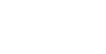Q – different

Original Capacitance: �< � = �<�     � = IJKLM I3

R

O

TI

C

AP

AC

�67 = �� = 05

234   (potential diff)

v = work/charge = J/c = volt

d

� = ��<= �� = ��<� � = �� cylindrical Capacitor:

� = �� = ��

2��<���7�6= 2��<�

V – different �Ou= 1�V+1�+1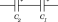V – same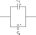�Ou = �V + �+

Energy stored in cap. �� = �+

2� = 12��+ = 12 ��

Increase cap. Prop. To �5NOP   = 2JKLM

23

K =2 ???? 2x increase in capacitance

�< = Q23� = Q2 � = ��< � = S3T (when constant Q) � = ��< = ��<�� = ���

Electric Energy Density: � = V+ ��<�+ = V+ ��+

C = Charge (Farads) Q = Current (coulombs) V = Voltage (volts)

���7�6

��5OmsNZt = V+ �<�+

Energy stored in an electric field: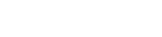�OPO). = V+ �� �5OmsNZt = V+ �<�+

Keep battery connected, insert Diel: Q increases,  V same ��OPO). = V+ ��+ = V+ ��<�+    Disconnect battery, insert diel:  Q same ��OPO). = �+

2� = �+

2��<

:

SR

O

T

SI

SE

R

Resistivity: � = ab (Ω*m)     Conductivity: VcDependent on material and temp. Resistance: � = cd_   (Ω,ohms)      More material = ↑ resis. Ohm’s Law (potential): � = �� (I = current)

series: Potential drops can  be different but Current =  same

parallel: V = same

Resistors (Series) I – Same � = �(�V + �+) = ��w �w = �V + �+ + �x     (effective resistance)

Resistors (parallel)  V – same

� = �V + �+ + �x � = ��V+��++��x �w= 1�V+1�+

1

If the electric field is strong enough, dielectric  Current – units = Amperes (A) � = 505Z = ���5�

A – area, n – num/vol(density), V-drift velocity, q – charge [ ��� = [ �� �� = �

� = ^_ = ���5 ???? current density

Electromotive Force: (EMF) units: V � = OmOz{t

)|6z{O  (ideal energy/unit of charge)

Kircheff’s Law (common  sense): when one branch  splits into 2: �NmZNN6P = �V + �+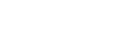Voltmeter (measures potential difference btwn its terminals) � = �� or �67 = ℰ − ��

Ammeter(measures current through it)

� = �

� + �

real sources contain internal resistance r Terminal Voltage: �67 = ℰ − �� Ideal emf: : ℰ = �� + �� � = } z~p

Voltage = change, current ≠change

:

S

Mag. Force on a moving charge (N)D

L

E

|�#| = �(�×�) = |�||�|�����

I

F

Particle is parallel to B: |�#| = 0

C

I

T

Particle is at angle to B: <use � from x

E

N

axis>

G

A

Particle is ⊥:  |�#| = |�||�|�

M

Right hand rule: hand points at V, fingers  curl in direction of B and point thumb out  ???? direction of F

Magnetic field lines ≠ “Lines of force” B

Helical motion

Component of velocity is ∥ and ⊥ to field

Mag. Force in Circle|�#| = ��� �) = ��+

��� = ��+

� => � = ��

��

Work = 0 b/c speed ≠ change. KE = constant

Motion of charged particles Charged particle always moves  with const. speed.

Electric Power: rate at which energy is  delivered to or extracted from a circuit  element. (Watts)� = �67� = �+ = 1o

p          E= Pt     W = Pt

� = ℰ� − �+� (ideal-rate of dis. Inside battery, pwr output)

Common Unit conv.

Mega      M      106

Kilo          k       103

Hecto      h        102

Deca        da    101

Deci         d       10-1

Centi        c      10-2

Milli          m     10-3

Micro        µ     10-6

Nano         n      10-9

�< = 8.85 x 10-12  F/m

Pitch: how far along particle moves in 1 rotation Cyclotron frequency:  # of rev./unit of time                                        Time for 1 orbit Time for 1 rev: � = +ÄÅ

u# � = ��

Page ExpiredIt looks like your free minutes have expired! Lucky for you we have all the content you need, just sign up here